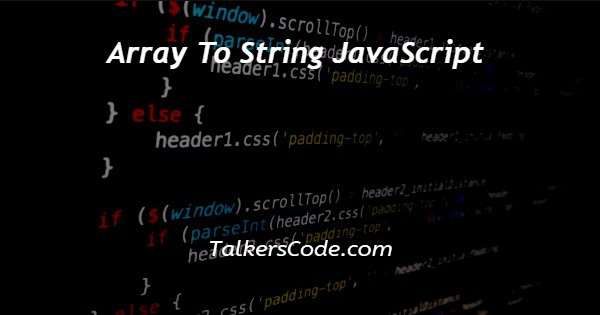# Array To String JavaScript

Last Updated : Jan 1, 2023In this tutorial we will show you the solution of array to string JavaScript, there are many ways with help of which we can convert array to string with help of JavaScript.

Let us see the example below for a better understanding with help of codes.

## Step By Step Guide On Array To String JavaScript :-

There are many ways with help of us to convert arrays to strings using JavaScript. The ways are:

1. With the help of the toString function
2. With the help of the join function

Let us see the examples given below of both of them for a quick view.

### By Using toString Function

```<p id="result"></p>
<script>
const colors = ["Red", "Yellow", "Green", "Blue"];
let string_here = colors.toString();
document.getElementById("result").innerHTML = string_here;
</script>
```

### By Using Join Function

```<p id="result"></p>
<script>
const cars = ["Mercedes", "Audi", "BMW", "Bantley"];
let resulted_string = cars.join();
document.getElementById("result").innerHTML = resulted_string;
</script>
```
1. As we here see that we today write only JavaScript codes in our example.
2. In previous tutorials, we already many times create a full code example in which we first create an HTML form with its basic structure. Inside that structure, we use HTML, head, title, body, and script tags.
3. In this example, as we see only, we write our JavaScript codes. The paragraph tag used here is only for display outputs. Now, let us understand our examples one by one.
4. In the first example, we see that a paragraph tag is created with an id. After that, we create our Script tag. A script tag is paired tag and is used to write scripts in between the opening and closing tag.
5. Here, we create a constant in which we store our array. inside the array, we store the values of colors in our first example. In the next line, we use our toString() function on our constant in which we store our array. The result of that function is stored inside a variable. Now, our job is to display the result so that we can see what is output and this is done using the paragraph tag which we created.
6. In our next example, that is done with the help of the join function. Here we have done the same process as the example of the toString function. But the difference here is that we used the join function instead of the toString function.
7. One thing to note here is that we can use the join function in many ways. As the join function is used join arrays can be done with the help of connectors. Instead of Commas as connectors, we can use any symbol like *, &, \$, +, etc., and any text to convert arrays to strings.

## Conclusion :-

At last, in conclusion, here we can say that with the help of this article we can understand how to convert an array to a string using JavaScript.

I hope this tutorial on array to string JavaScript helps you and the steps and method mentioned above are easy to follow and implement.